SBAS562B April   2012  – December 2019

PRODUCTION DATA.

1. Features
2. Applications
3. Description
1.     Device Images
4. Revision History
5. Pin Configuration and Functions
6. Specifications
7. Detailed Description
8. Application and Implementation
1. 8.1 Application Information
2. 8.2 Typical Applications
1. 8.2.1 The AMC1100 in Frequency Inverters
2. 8.2.2 The AMC1100 in Energy Metering
9. Power Supply Recommendations
10. 10Layout
11. 11Device and Documentation Support
1. 11.1 Device Support
1. 11.1.1 Device Nomenclature
1. 11.1.1.1 Isolation Glossary
2. 11.2 Documentation Support
4. 11.4 Support Resources
6. 11.6 Electrostatic Discharge Caution
7. 11.7 Glossary
12. 12Mechanical, Packaging, and Orderable Information

• DUB|8
• DWV|8
• DUB|8

### 6.11 Typical Characteristics

At VDD1 = VDD2 = 5 V, VINP = –250 mV to +250 mV, and VINN = 0 V, unless otherwise noted.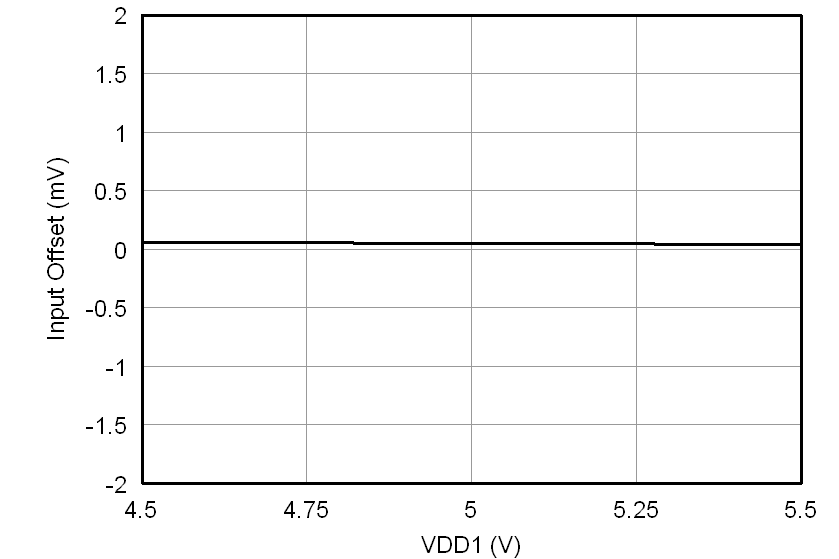Figure 3. Input Offset vs High-Side Supply Voltage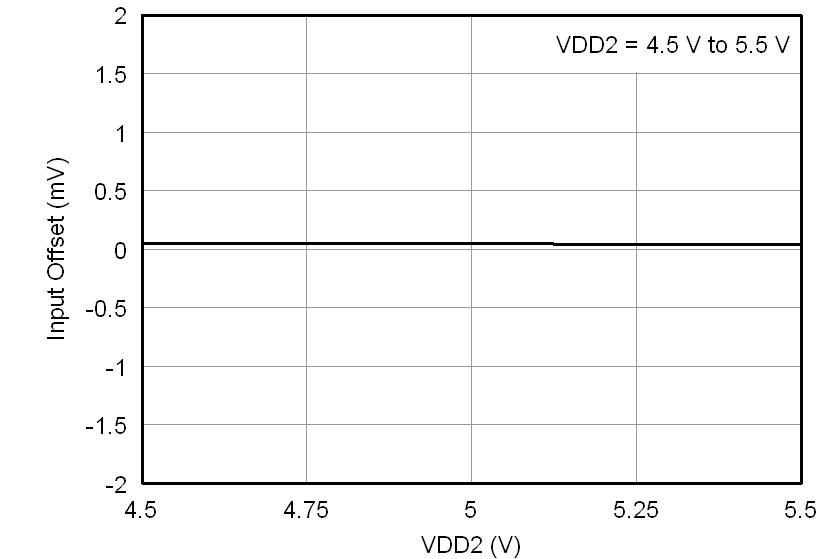Figure 5. Input Offset vs Low-Side Supply Voltage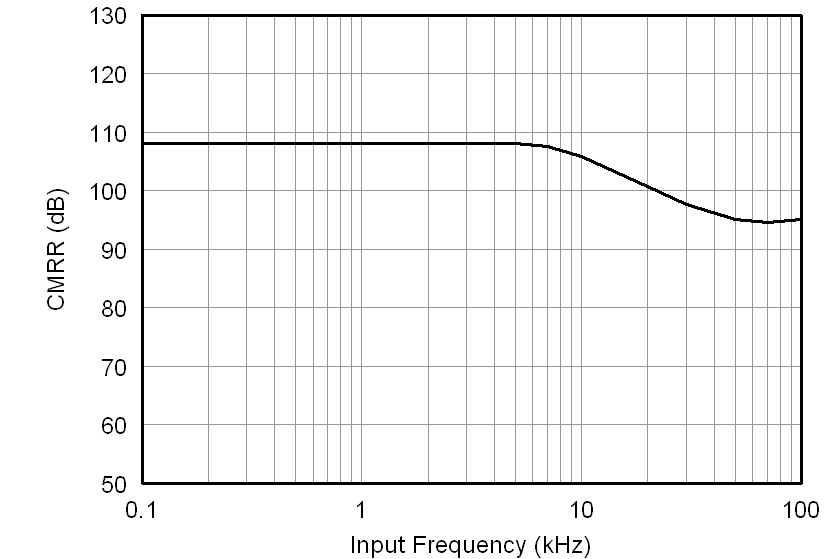Figure 7. Common-Mode Rejection Ratio vs
Input Frequency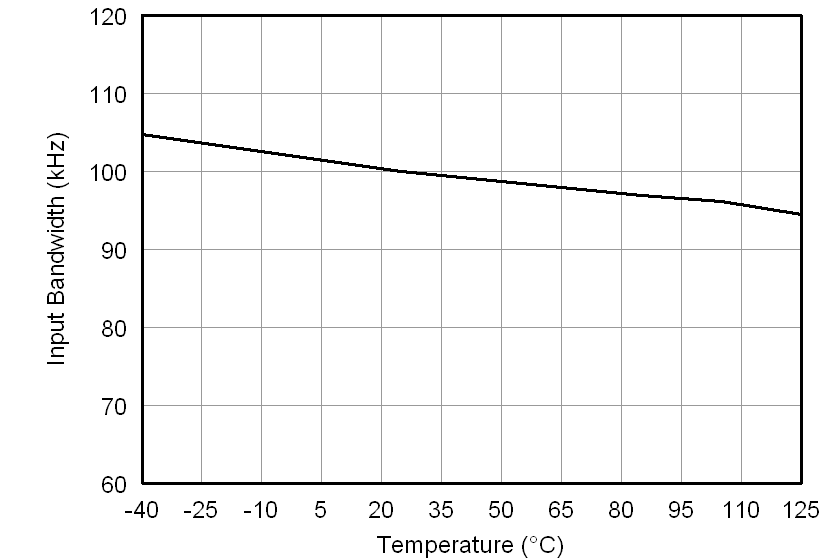Figure 9. Input Bandwidth vs Temperature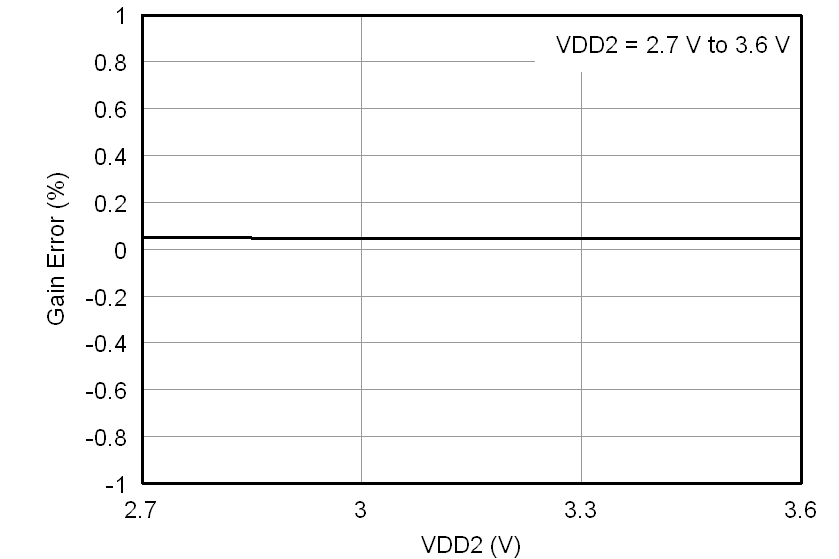Figure 11. Gain Error vs Low-Side Supply Voltage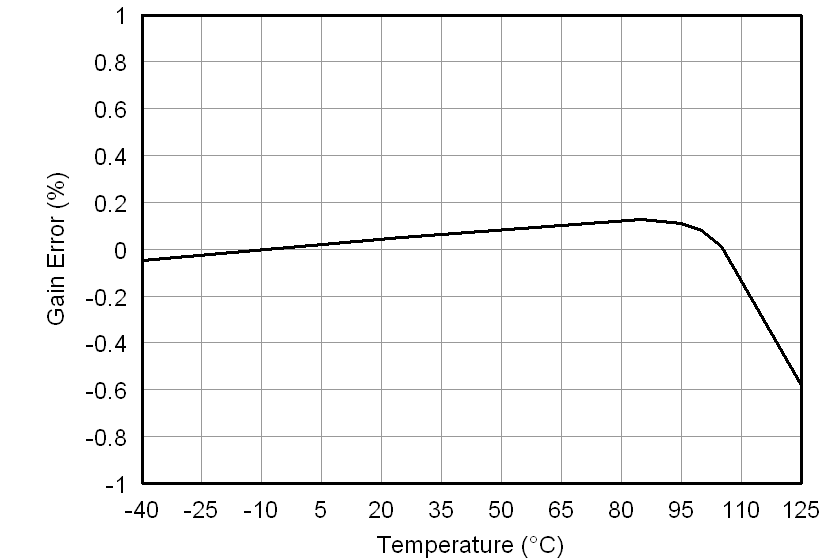Figure 13. Gain Error vs Temperature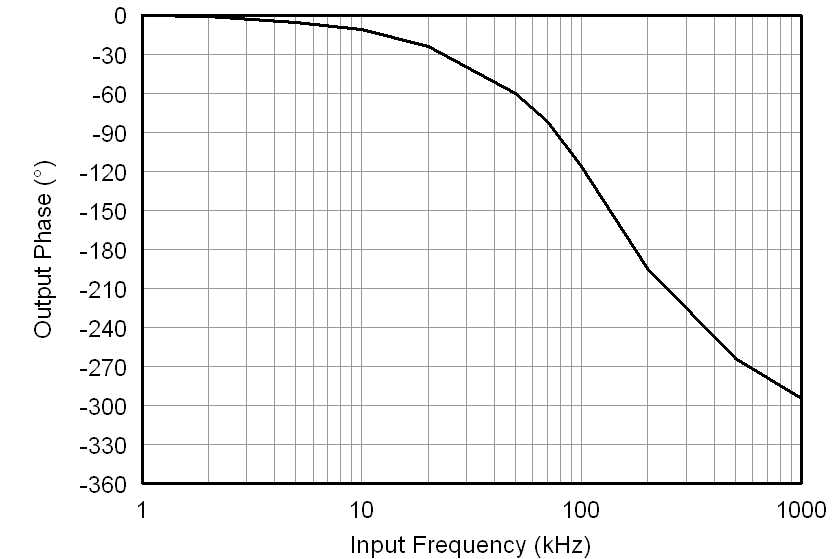Figure 15. Output Phase vs Input Frequency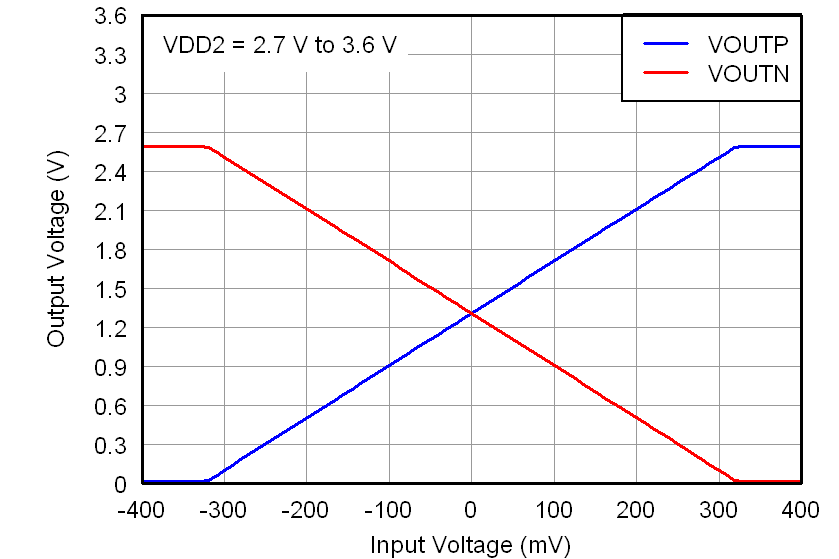Figure 17. Output Voltage vs Input Voltage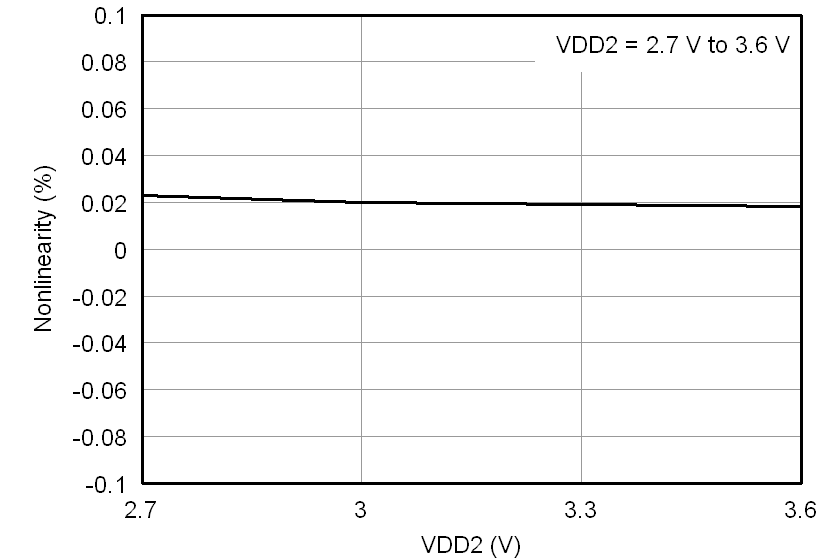Figure 19. Nonlinearity vs Low-Side Supply Voltage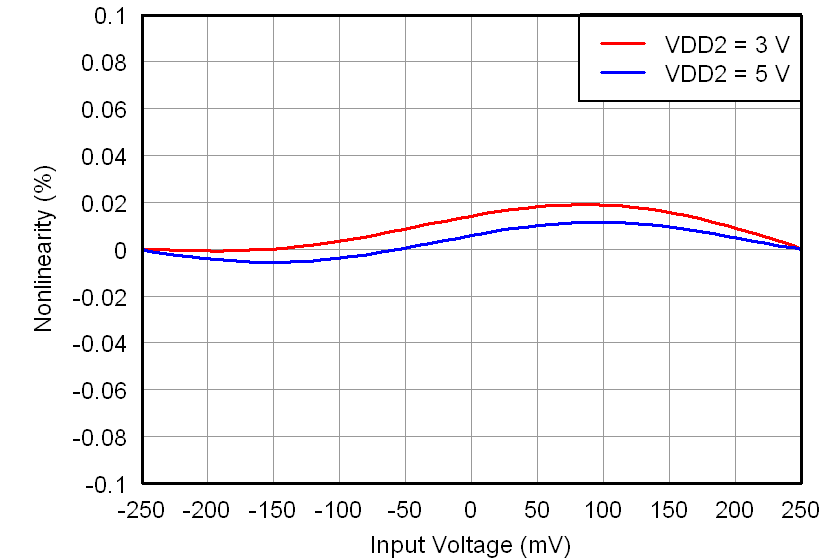Figure 21. Nonlinearity vs Input Voltage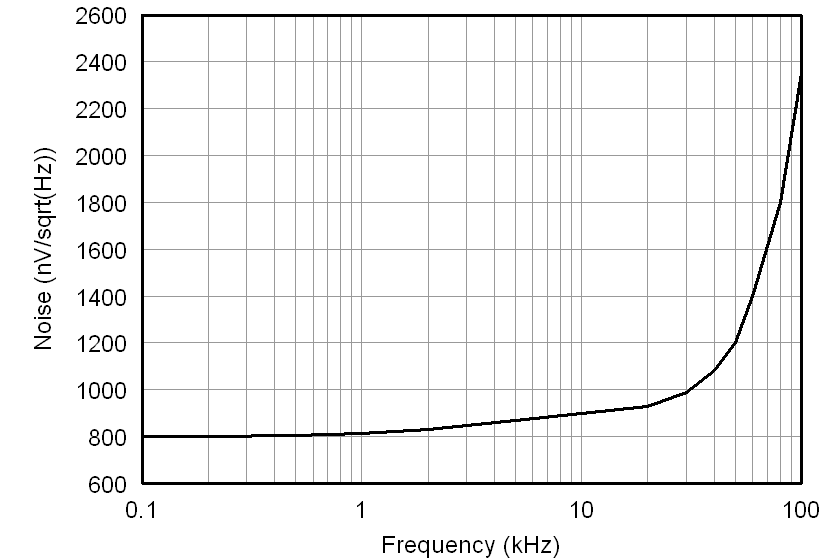Figure 23. Output Noise Density vs Frequency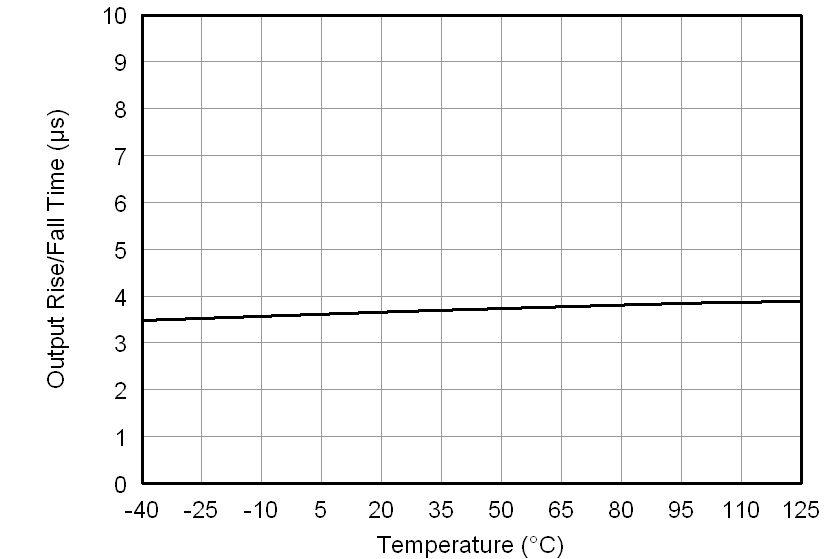Figure 25. Output Rise and Fall Time vs Temperature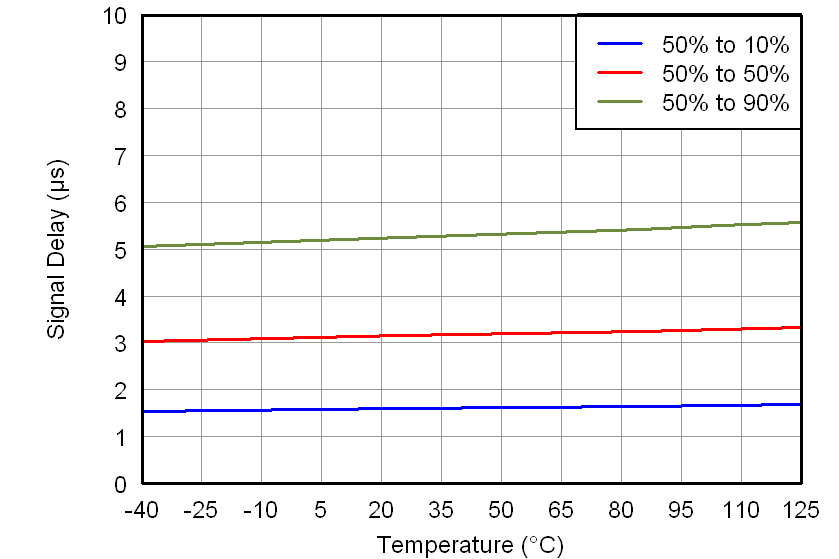Figure 27. Output Signal Delay Time vs Temperature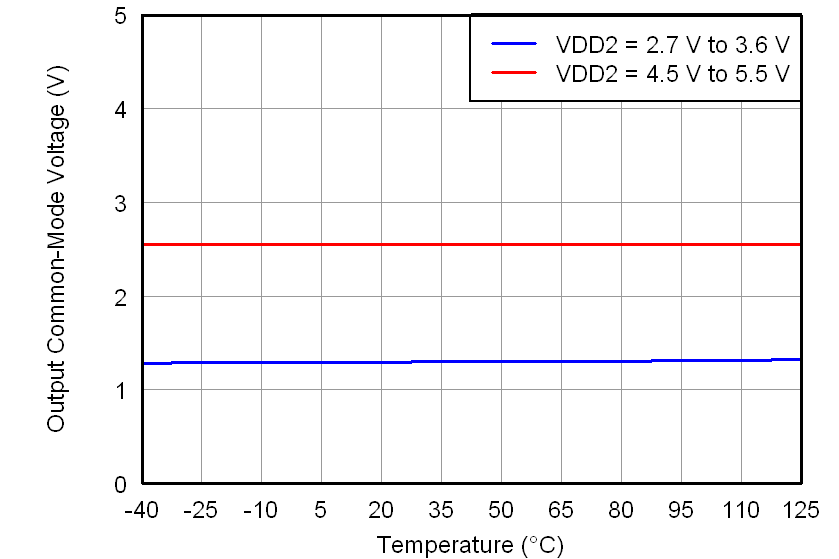Figure 29. Output Common-Mode Voltage vs Temperature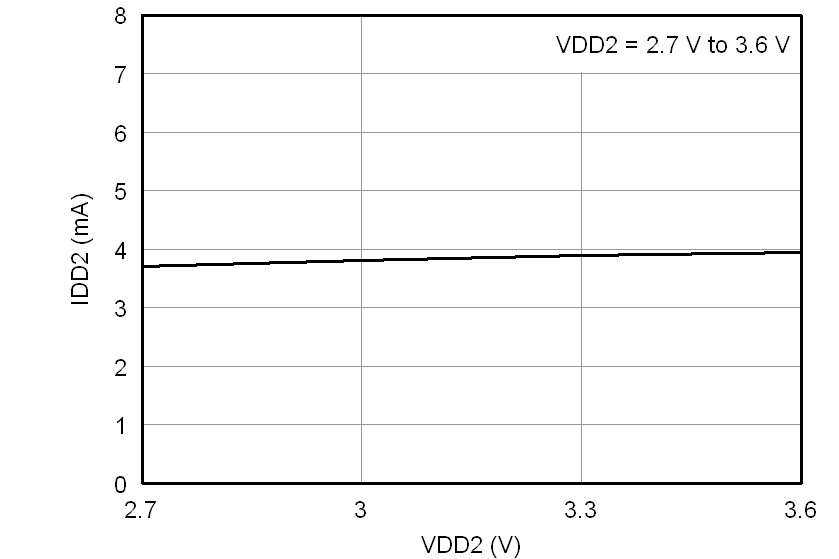Figure 31. Low-Side Supply Current vs
Low-Side Supply Voltage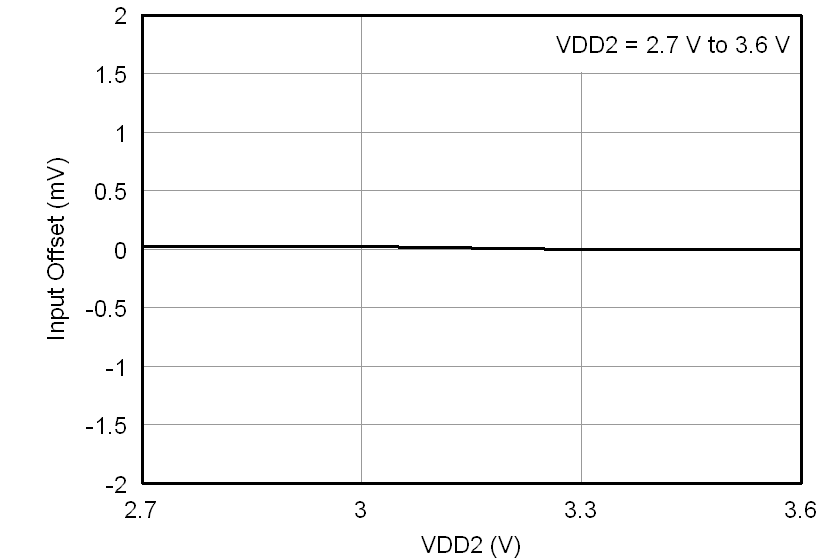Figure 4. Input Offset vs Low-Side Supply Voltage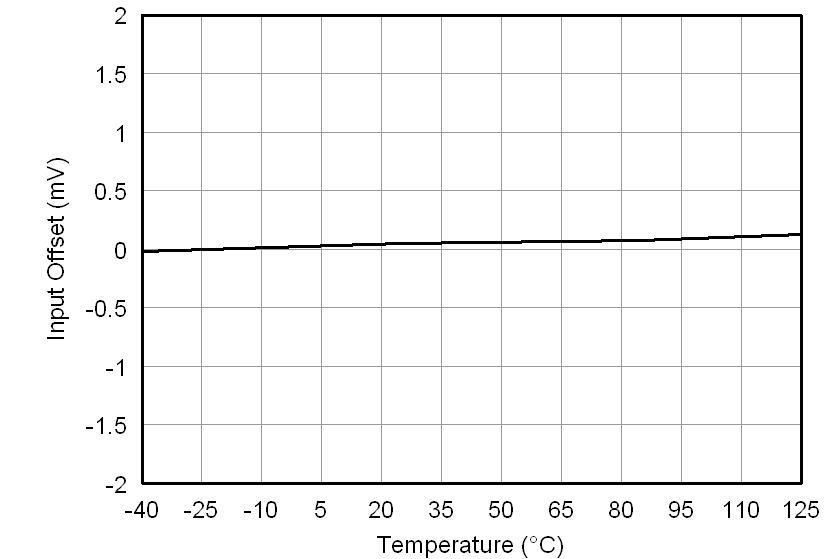Figure 6. Input Offset vs Temperature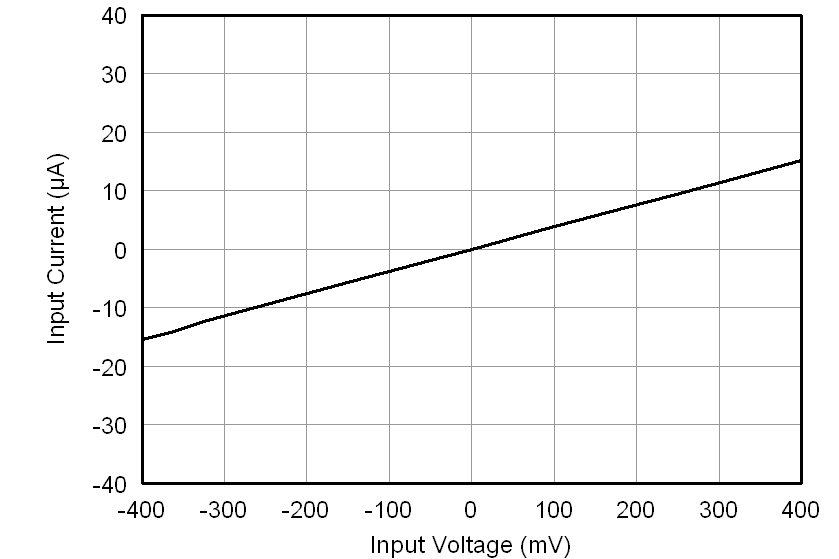Figure 8. Input Current vs Input Voltage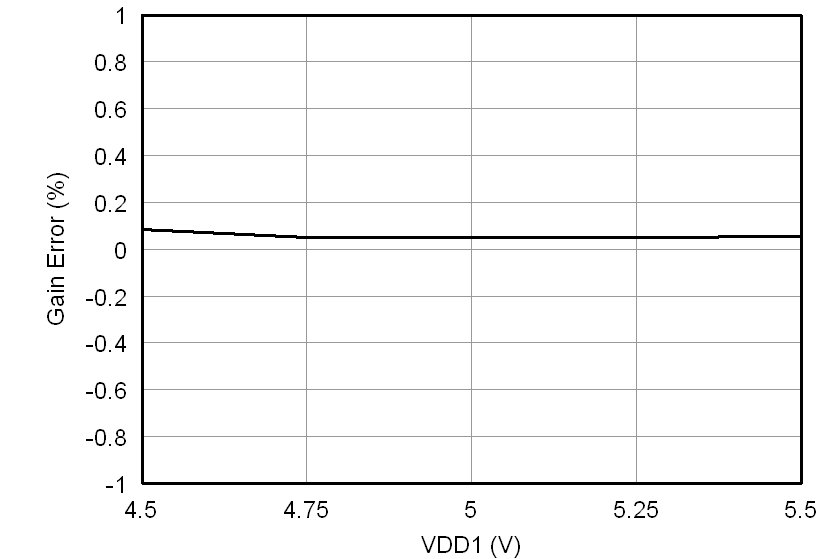Figure 10. Gain Error vs High-Side Supply Voltage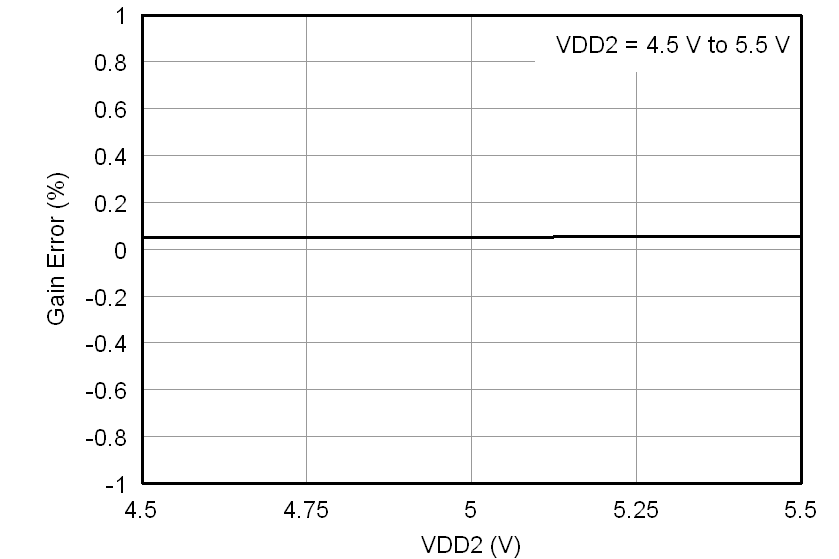Figure 12. Gain Error vs Low-Side Supply Voltage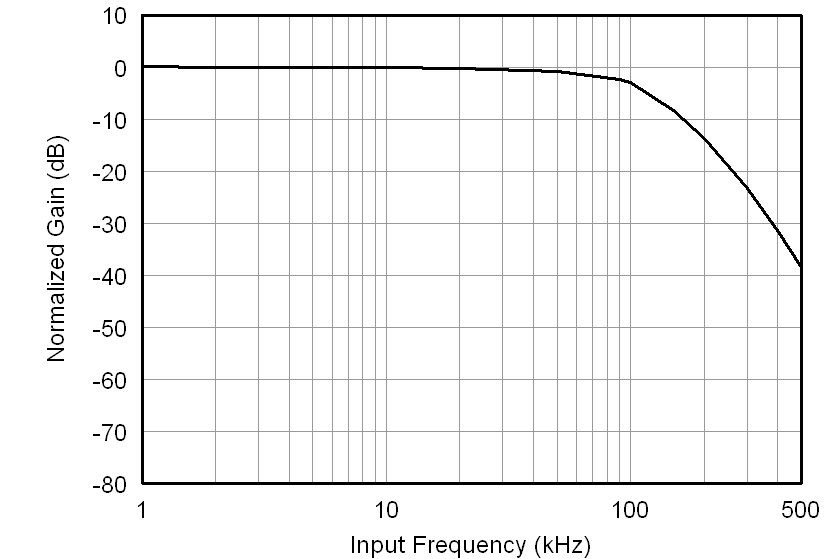Figure 14. Normalized Gain vs Input Frequency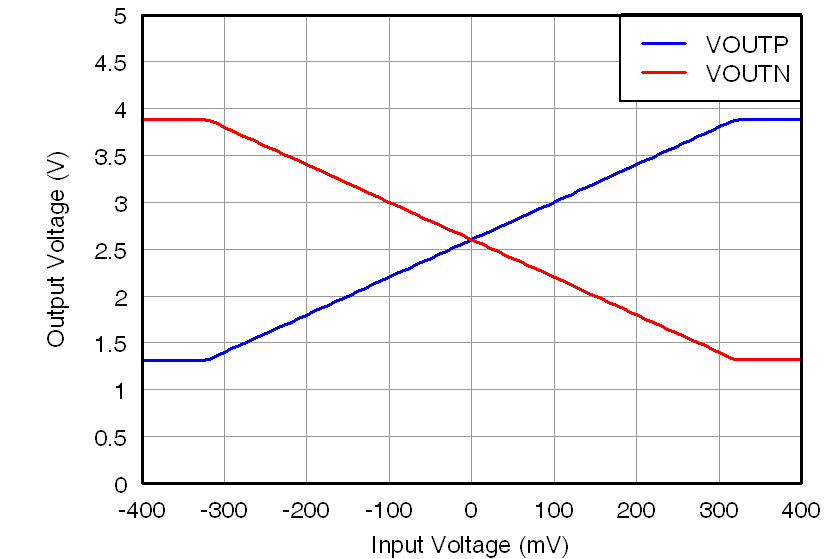Figure 16. Output Voltage vs Input Voltage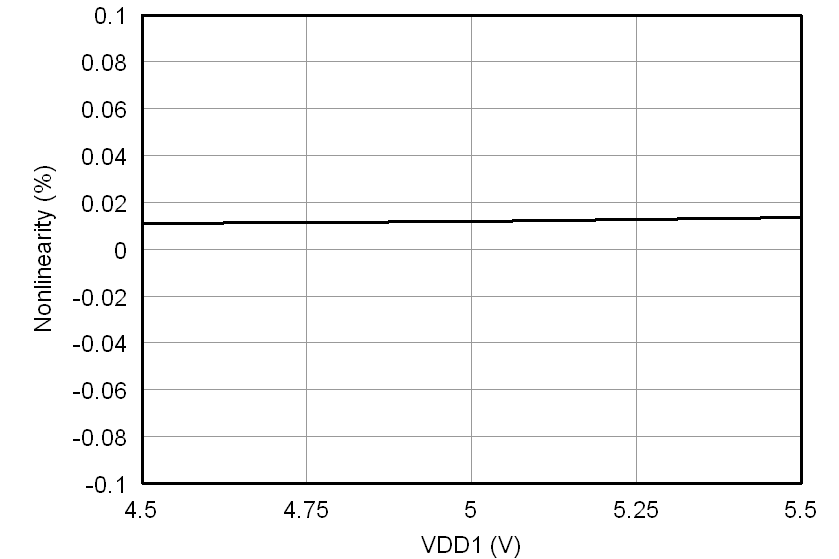Figure 18. Nonlinearity vs High-Side Supply VoltageFigure 20. Nonlinearity vs Low-Side Supply Voltage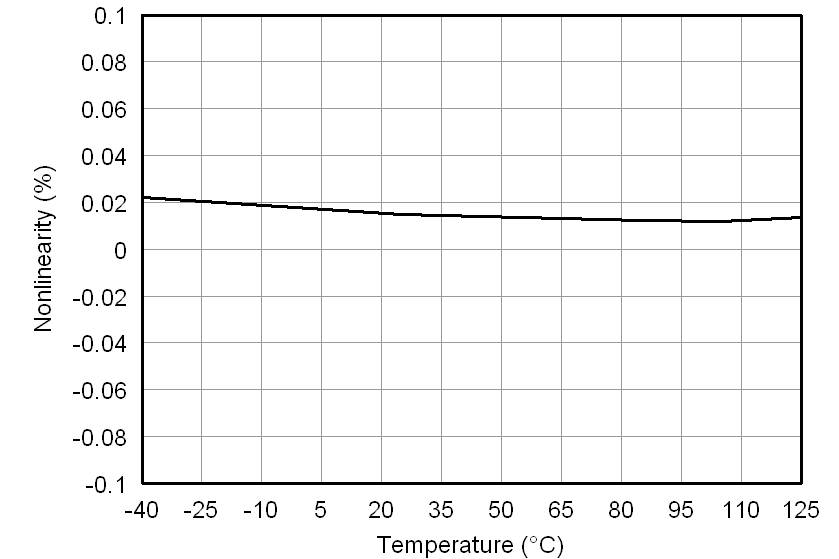Figure 22. Nonlinearity vs Temperature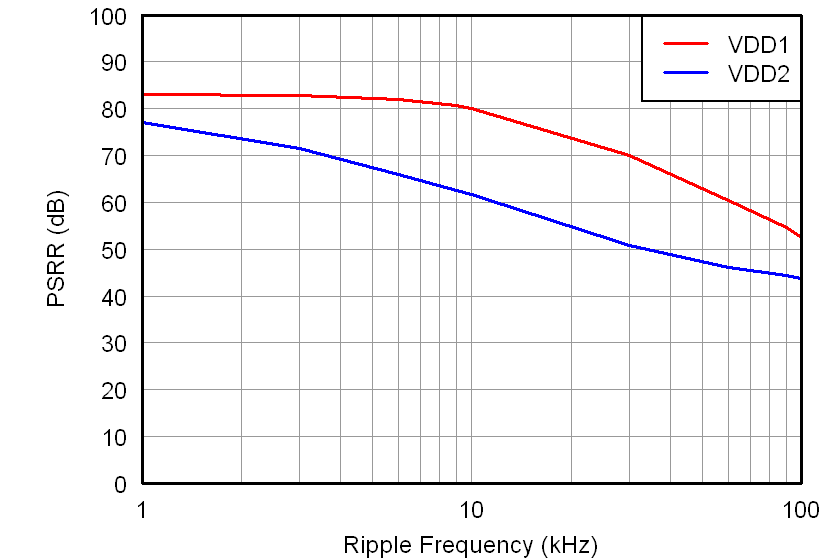Figure 24. Power-Supply Rejection Ratio vs
Ripple Frequency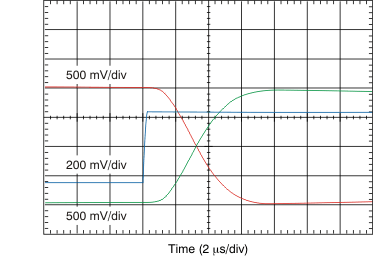Figure 26. Full-Scale Step Response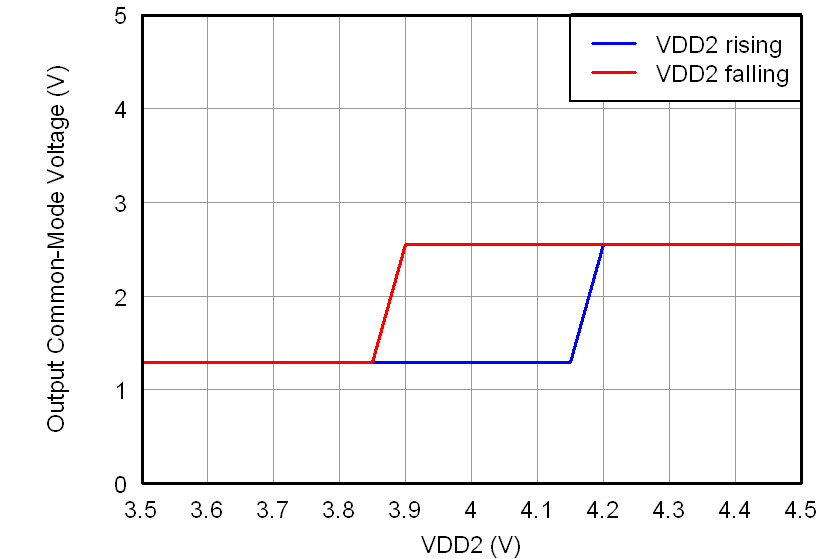Figure 28. Output Common-Mode Voltage vs
Low-Side Supply Voltage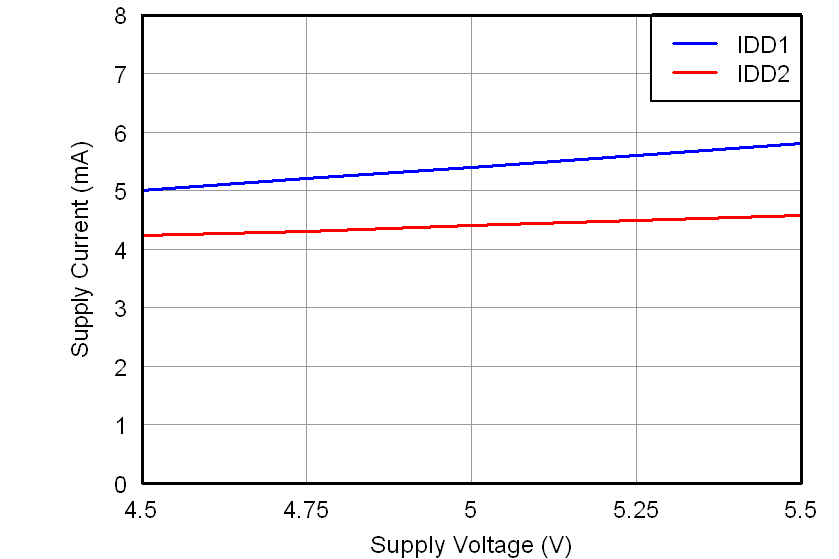Figure 30. Supply Current vs Supply Voltage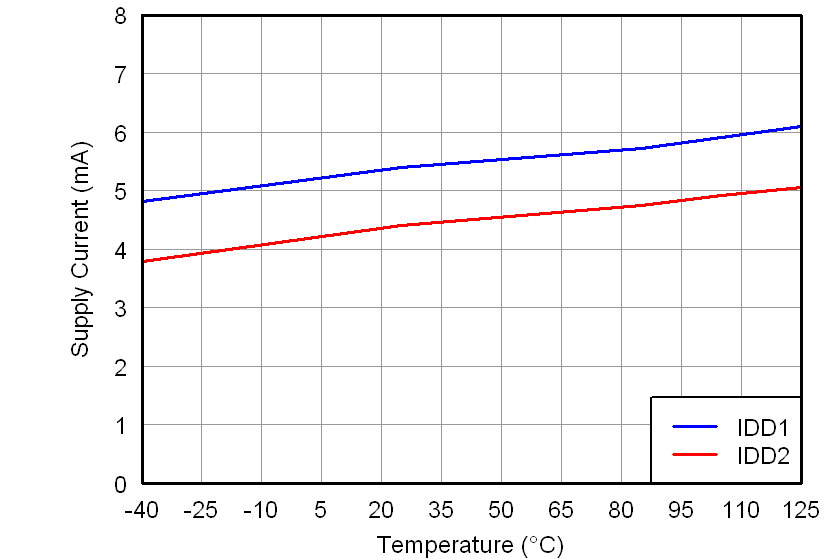Figure 32. Supply Current vs Temperature